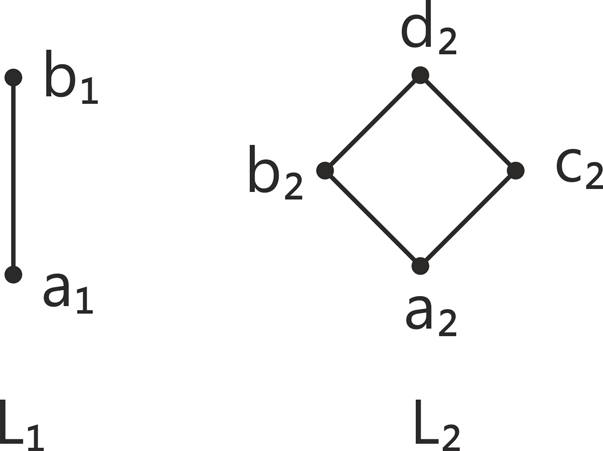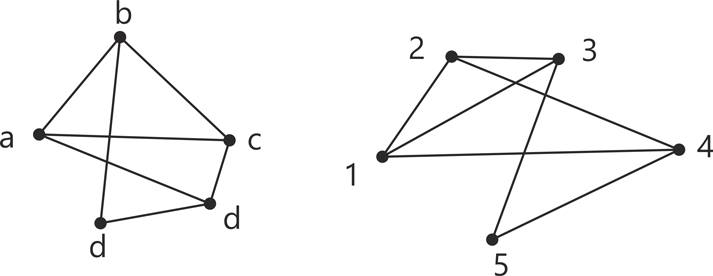MORE IN Discrete Structures
MU Computer Engineering (Semester 3)
Discrete Structures
December 2013
Total marks: --
Total time: --
INSTRUCTIONS
(1) Assume appropriate data and state your reasons
(2) Marks are given to the right of every question
(3) Draw neat diagrams wherever necessary

1 (a) Prove that in a full binary tree with n vertices, the number of pendant vertices is (n+1)/2
4 M
1 (b) Let G be the set of rational numbers other than 1. let define an operation * on G by a*b=a+b-ab for all a,b∈ G. prove that (G,*) is a group.
6 M
1 (c) Find the number of integers between 1 and 1000 which are
(i) Divisible by 2,3 or 5
(ii) Divisible by 3 only but not by 2 nor by 5.
5 M
1 (d) Find all solutions of the recurrence relation
an=5an-1 + 6an-2 + 7n
5 M

2 (a) Prove by mathematical induction xn - yn is divisible by x-y.
4 M
2 (b) Let m be the positive integers greater that 1. show that the relation R={ (a,b)|a=b (mod m)}, i.e. aRb if and only if m divides a-b is an equivalance relation on the set of integers.
6 M
2 (c) Let s= {1, 2, 3, 4} and A=S x S. define the following relation :- R on A : (a,b) R(a', b) if and only if a+b=a'+b.
(i) show that R is an equivalance relation.
(ii) compute A/R.
6 M
2 (d) If f : A → B be both one-to-one and onto, then prove that f1 : B → A is also both one-to-one and onto.
4 M

3 (a) Consider an equilateral triangle whose sides are of length 3 units. If ten points are chosen lying on or inside the triangle, then show that at least two of them are no more than 1 unit and onot.
5 M
3 (b) Let L1 and L2 be lettices shown below :- Draw the Hasse diagram of L1 x L2 with product partial order :7 M
3 (c) Let A={a,b,c}. Show that (P(A), ⊆) is a poset. Draw its hasse diagram. P(A) is the power set of A.
4 M
3 (d) How many vertices are necessary to construct a graph with exactly 6 edges in which each vertex is of degree 2.
4 M

4 (a) Show that is every element in a group is its own inverse, then the group must be abelian.
4 M
4 (b) If (G, *) is an abelian group, then for all a, b ∈ G, prove that by mathematical induction (a*b)n=an * bn.
5 M
4 (c) If f is a homorphism from a commutative group (S, *) to another group (T, *'), then prove that (T,*') is also commutative.
4 M
4 (d) Consider the (3,5) group encoding function
e: B3 → B5 defined by
e(000)=00000 e(100)=10011
e(001)=00110 e(101)=10101
e(010)=01001 e(110)=11010
e(011)=01111 e(111)=11100
Decode the following words relative to maximum likelyhood decoding function
(i) 11001 (ii)01010 (iii) 00111
7 M

5 (a) Find the generation function for the following sequence
1, 2, 3, 4, 5, 6,. . .
5 M
5 (b) Solve the recurence relation ar=3ar-1+2, r≥1 with a0=1 using generation function.
6 M
5 (c) Show that the following graphs are isomorphic. :5 M
5 (d) use the laws of logic to show that
[ (p⇒q)¬q]⇒¬p is a tautology.
4 M

More question papers from Discrete Structures